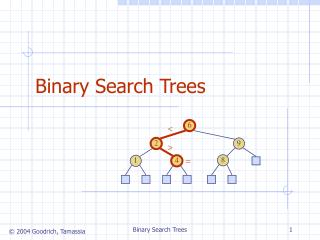DownloadDownload PresentationBinary Search Trees

# Binary Search Trees

Télécharger la présentation## Binary Search Trees

- - - - - - - - - - - - - - - - - - - - - - - - - - - E N D - - - - - - - - - - - - - - - - - - - - - - - - - - -
##### Presentation Transcript

1. Binary Search Trees 6 < 2 9 > = 8 1 4 Binary Search Trees

2. Ordered Dictionaries • Keys are assumed to come from a total order. • New operations: • first(): first entry in the dictionary ordering • last(): last entry in the dictionary ordering • successors(k): iterator of entries with keys greater than or equal to k; increasing order • predecessors(k): iterator of entries with keys less than or equal to k; decreasing order Binary Search Trees

3. Binary Search • Binary search can perform operation find(k) on a dictionary implemented by means of an array-based sequence, sorted by key • similar to the high-low game • at each step, the number of candidate items is halved • terminates after O(log n) steps • Example: find(7) 0 1 3 4 5 7 8 9 11 14 16 18 19 m h l 0 1 3 4 5 7 8 9 11 14 16 18 19 m h l 0 1 3 4 5 7 8 9 11 14 16 18 19 m h l 0 1 3 4 5 7 8 9 11 14 16 18 19 l=m =h Binary Search Trees

4. A search table is a dictionary implemented by means of a sorted sequence We store the items of the dictionary in an array-based sequence, sorted by key We use an external comparator for the keys Performance: find takes O(log n) time, using binary search insert takes O(n) time since in the worst case we have to shift n/2 items to make room for the new item remove take O(n) time since in the worst case we have to shift n/2 items to compact the items after the removal The lookup table is effective only for dictionaries of small size or for dictionaries on which searches are the most common operations, while insertions and removals are rarely performed (e.g., credit card authorizations) Search Tables Binary Search Trees

5. A binary search tree is a binary tree storing keys (or key-value entries) at its internal nodes and satisfying the following property: Let u, v, and w be three nodes such that u is in the left subtree of v and w is in the right subtree of v. We have key(u)key(v) key(w) External nodes do not store items (for now) An inorder traversal of a binary search trees visits the keys in increasing order 6 2 9 1 4 8 Binary Search Trees Binary Search Trees

6. Search AlgorithmTreeSearch(k, v) ifT.isExternal (v) returnv if k<key(v) returnTreeSearch(k, T.left(v)) else if k=key(v) returnv else{ k>key(v) } returnTreeSearch(k, T.right(v)) • To search for a key k, we trace a downward path starting at the root • The next node visited depends on the outcome of the comparison of k with the key of the current node • If we reach a leaf, the key is not found and we return null • Example: find(4): • Call TreeSearch(4,root) 6 < 2 9 > = 8 1 4 Binary Search Trees

7. Insertion 6 < • To perform operation insert(k, o), we search for key k (using TreeSearch) • Assume k is not already in the tree, and let w be the leaf reached by the search • We insert k at node w and expand w into an internal node • Example: insert 5 2 9 > 1 4 8 > w 6 2 9 1 4 8 w 5 Binary Search Trees

8. Deletion 6 < • To perform operation remove(k), we search for key k • Assume key k is in the tree, and let v be the node storing k • If node v has a leaf child w, we remove v and w from the tree with operation removeExternal(w), which removes w and its parent • Example: remove 4 2 9 > v 1 4 8 w 5 6 2 9 1 5 8 Binary Search Trees

9. Deletion (cont.) 1 v • We consider the case where the key k to be removed is stored at a node v whose children are both internal • we find the internal node w that follows v in an inorder traversal • we copy key(w) into node v • we remove node w and its left child z (which must be a leaf) by means of operation removeExternal(z) • Example: remove 3 3 2 8 6 9 w 5 z 1 v 5 2 8 6 9 Binary Search Trees

10. Performance • Consider a dictionary with n items implemented by means of a binary search tree of height h • the space used is O(n) • methods find, insert and remove take O(h) time • The height h is O(n) in the worst case and O(log n) in the best case Binary Search Trees